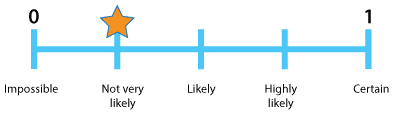### Probabilities range from 0 to 1

Probability is a measure of the likeliness or chance of possibilities in a situation. It is not possible to have a length or area or weight or height less than zero. In the same way, probability cannot be below 0. You can't have less than zero chance of something happening.

#### Zero probabilityDetailed description

Can probability be equal to 0? Yes, if something is not possible at all, there is no chance it will happen. Its chance or probability of happening is 0.

There is zero probability of the spinner on the right landing on black. There is zero probability that a 7 can be rolled on a normal six-sided die on one roll.

#### Probability equal to 1

If something is certain to happen it has probability of 1. So the probability of spinning orange or red or green or blue on one spin of the spinner above is 1, it is certain that you will spin one of them.

#### Probability between 0 and 1

If an event is neither certain nor impossible, then the probability of that event occurring is somewhere between 0 and 1. A very, very small chance of something happening is not a zero chance. On the scale below, we can show that there may be a very, very small chance that it will snow in Brisbane but it's not absolutely impossible, so we say that it is not very likely and assign a probability of just a little more than zero.Detailed description

An almost certain probability is not equal to 1. Whilst it is almost certain that my car will start when I leave for home today, there is still a small chance it will not. So, we would say that it is highly likely that my car will start and assign a probability of just a little less than 1.